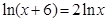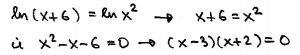# Math Maturity – Miroslav Lovric

## Math Maturity’s Docs▸Math Maturity - Miroslav Lovric

You are currently viewing a revision titled "Math Maturity - Miroslav Lovric", saved on February 7, 2019 at 5:00 am by sasha-admin
Title
Math Maturity - Miroslav Lovric
Content
In a discussion during the conference, the term “math maturity” was mentioned.  We agreed that it is a poorly defined term and could mean lots of different things. In my view, math maturity refers to the growth of an individual as a mathematician, and is based on their experiences, insights and reflection. Developed by repeated exposure to mathematics, math maturity includes (but is not limited to) ability to read and understand math notation and work with math symbols; capacity to handle abstract ideas, and to generate abstractions; ability to ask right questions; ability to reason logically and to produce a coherent mathematical narrative. Instead of going into details here, I will illustrate what I mean in a couple of examples. In my experience (and that of my colleagues), even our better math students, after taking a number of math courses, do not possess those extra qualities that would qualify them as math mature. Elementary example. When we ask students to solve the equationwe see the usual sequence of stepsfrom which many conclude that there are two solutions, x=3 and x=-2. Very few students note that x cannot be negative. (Math maturity: no matter what equation you are solving, check whether or not solutions make sense.) Advanced example, coming from my third-year problem solving course. A typical student in this course has taken a minimum of 6-7 university math courses. To start, I ask my students to write a code (in Python) to generate a list of first one million prime numbers. Pointing that this is really cool and exciting (as only 20 years ago producing such a list would take lot more effort and time), students work on a problem sheet, which suggests ideas they can investigate. The last question asks them to identify interesting facts about prime numbers, and/or the prime number counting function, that they discovered on their own. Here is a sample of their completeanswers: “The numbers increase exponentially” “There are less and less prime numbers” “All prime numbers are relatively prime” “The only even prime number is 2; no prime greater than 5 ends with 5. I googled these” I do not think that anyone would call these math-mature answers.

## 3 thoughts on “About Math Maturity”

1.Carmen Bruni says:
With regard to your first point, I’ve actually had many discussions with people about the logarithmic solution you posted. We as mathematicians are extremely sloppy. Really when we say something like “Solve x^2 – x – 6 = 0” We actually want an answer to A real number x satisfies x^2 – x – 6 = 0 if and only if ______ where the blank is what we want to fill in. To be logically correct we should *always* substitute in our last answer into the original equation. Otherwise, the solution x^2 – x – 6 = 0 x(x^2 – x – 6) = 0 x(x-3)(x+2) = 0 x = -2,0,3 is a valid sequence of steps from top to bottom (but of course not from bottom to top). I suspect this subtly is lost on a lot of mathematics educators at all levels and really only comes up when you’re really thinking about it in a proofs class. I have no idea what the right way to correct this is. I’ve written a small note that I haven’t shared with many people before now (I’ll attach a link below) but I’m interested in knowing how other people resolve this, especially in their first year proofs class! https://cs.uwaterloo.ca/~cbruni/pdfs/NoteOnEquationProofs.pdf Thanks for the post!
1.cbruni says:
With regard to your first point, I’ve actually had many discussions with people about the logarithmic solution you posted. We as mathematicians are extremely sloppy. Really when we say something like “Solve x^2 – x – 6 = 0” We actually want an answer to A real number x satisfies x^2 – x – 6 = 0 if and only if ______ where the blank is what we want to fill in. To be logically correct we should *always* substitute in our last answer into the original equation. Otherwise, the solution x^2 – x – 6 = 0 x(x^2 – x – 6) = 0 x(x-3)(x+2) = 0 x = -2,0,3 is a valid sequence of steps from top to bottom (but of course not from bottom to top). I suspect this subtly is lost on a lot of mathematics educators at all levels and really only comes up when you’re really thinking about it in a proofs class. I have no idea what the right way to correct this is. I’ve written a small note that I haven’t shared with many people before now (I’ll attach a link below) but I’m interested in knowing how other people resolve this, especially in their first year proofs class! https://cs.uwaterloo.ca/~cbruni/pdfs/NoteOnEquationProofs.pdf Thanks for the post!
2.Judy Mendaglio says:
When trying to define and assess “mathematical maturity”, it may be best to be very targeted and specific to be able to identify how the lack of maturity manifests itself, with eventual hopes of remediating this problem. – ability to read and understand math notation (Log problem — students could do this) – ability to work with math symbols (Log problem — students could do this) – capacity to handle abstract ideas (This may be where it starts to break down, although they seem to be able to handle abstract ideas — it is recognizing that the abstract ideas have concrete constraints that fails?) – generate abstractions (This will certainly mean different things to different people) – ability to ask right questions (This is a real issue and it is certainly not limited to mathematics. From the time they enter school, they are exposed to others’ (teachers’, textbooks’) questions and their own natural curiosity is no longer valued, to the extent that , by the time the reach middle school, their concept of a good math question is “Which formula is the right one for this?”. There is a fairly recent recognition that students now need to be taught how to ask meaningful questions.) – ability to reason logically (Log problem — students think this is what they are doing — they are applying all the steps in a logical sequence!) – to produce a coherent mathematical narrative (Ah! Here is where it all fails! Coherence requires making connections between the conceptual and procedural and, well, that is not happening. It seems the procedural is all good, but the concepts don’t seem to have made much of an impression on them.)
Excerpt

Old New Date Created Author Actions
February 7, 2019 at 5:00 am sasha-admin
February 7, 2019 at 4:58 am sasha-admin